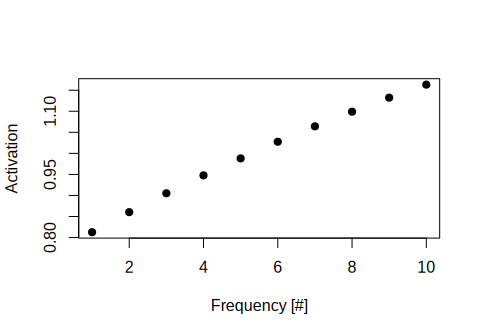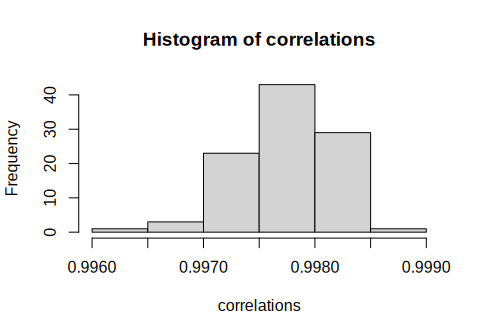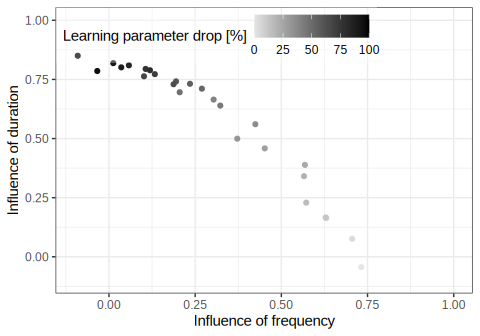# A too short introduction to PASS-T with the passt R package

#### 2021-05-03

The passt package is an R implementation of the Probability ASSociator Time (PASS-T) model, an artificial neural network designed to explain how humans make judgments of frequency and duration (Titz & Sedlmeier, 2019). The package was developed with two purposes in mind: (1) to provide a simple way to reproduce simulation results in judgments of frequency and duration as described in Titz & Sedlmeier (2019), and (2) to explore the PASS-T model by allowing users to run their own individual simulations.

The general idea of the original PASS model (Sedlmeier, 1999, 2002) is that information about the frequency of events is naturally incorporated in artificial neural networks. If an object is presented repeatedly, the weights of the network change systematically. This way, the network is able to deduce the frequency of occurrence of events based on the final weights. Put simply, the artificial neural network produces a higher activation the more often a stimulus is presented.

As an extension of the PASS model, PASS-T is also able to process the presentation time of the stimuli. One can define how often and how long different objects are presented and test whether the network is able to make valid judgments of frequency and duration in retrospect. The principal aim of PASS-T is to explain empirical results that are found in studies with humans (Titz, Burkhardt, & Sedlmeier, 2019). Specifically, two empirical results are quite robust: (1) the correlation between exposure frequency and judgment is usually larger than between exposure duration and judgment (sometimes termed frequency primacy); and (2) the amount of attention that participants pay to the stimuli moderates effect sizes. These findings have been of some interest in cognitive psychology in the last decade (Betsch, Glauer, Renkewitz, Winkler, & Sedlmeier, 2010; Titz et al., 2019; Titz & Sedlmeier, 2019; Winkler, 2009; Winkler, Glauer, Betsch, & Sedlmeier, 2015), although its roots go back half a century (Cooper & Pantle, 1967; Hintzman, 1970; Hintzman, Summers, & Block, 1975) and touch upon different research areas such as memory, heuristics, and perception of magnitudes. PASS-T can be seen as a summary of some of this research, cast into a formal model. The R package passt is the first concrete implementation of PASS-T to make the model available to a wider audience.

# Using passt

There are only two functions of passt that you will need to use. The first function is run_sim, which runs several simulations with specific parameters and returns the final output activation for each input pattern. The second is run_exp, which aggregates the data and gives effect sizes for each simulation run. Let us first look at run_sim.

Since PASS-T extends the PASS model, PASS-T should be able to produce all the results that PASS can produce. The simplest PASS simulation is a type of counter: an artificial neural network sensitive only to frequency information.

## A simple counter

Let us first load the package and set a seed so that the results are reproducible:

library(passt)
set.seed(20191015)
sim1 <- run_sim(patterns = diag(10), frequency = 1:10, duration = 10:1,
lrate_onset = 0.05, lrate_drop_time = 2, lrate_drop_perc = 0)

Some explanation is necessary: patterns is a matrix of input patterns that the network will process. Here we will use orthogonal stimuli to avoid any interference on the input level. The function diag will create an identity matrix so that every input pattern has only one active unit. This active unit will be unique for each pattern. You can also use other stimulus patterns as long as the activation for each unit is either 0 or 1.

Next, we specify how often the ten patterns should be shown and the duration of each presentation. The first pattern will be shown once for 10 s, the second pattern will be shown twice for 9 s each (a total duration of 18 s), and so on. You can also use other arrangements for a simple counter.

Finally, we set the learning rate parameters: the initial learning rate (lrate_onset) is 0.05, but after 2 s (lrate_drop_time) it will drop to 0 (lrate_drop_perc). This parameter selection is crucial for a counter because only the first two seconds of a stimulus presentation are processed by the network.

The function run_sim returns a list with three elements, of which output is the most interesting. Note that, by default, 100 simulations are run (you can change this with the parameter n_runs). We will only look at the first couple of simulations:

head(sim1$output) ## [,1] [,2] [,3] [,4] [,5] [,6] [,7] ## [1,] 0.8134030 0.8623727 0.9090286 0.9526607 0.9893114 1.027876 1.065307 ## [2,] 0.8161057 0.8618859 0.9028135 0.9479106 0.9905695 1.024340 1.062587 ## [3,] 0.8117953 0.8602871 0.9049667 0.9490695 0.9895403 1.027940 1.061659 ## [4,] 0.8148240 0.8633805 0.9009140 0.9490142 0.9884823 1.026991 1.061192 ## [5,] 0.8114915 0.8611520 0.9011725 0.9449056 0.9886680 1.027973 1.067783 ## [6,] 0.8177491 0.8598771 0.9061970 0.9493045 0.9831946 1.031035 1.059464 ## [,8] [,9] [,10] ## [1,] 1.093939 1.125975 1.160127 ## [2,] 1.098260 1.132112 1.163416 ## [3,] 1.097431 1.134466 1.162845 ## [4,] 1.100671 1.128499 1.166032 ## [5,] 1.103455 1.132242 1.161158 ## [6,] 1.102026 1.132638 1.158515 The output gives the sum of the activity of all output units for the specific patterns for each simulation run. Each row corresponds with one simulation and each column with one input pattern. Sometimes the output activation is referred to as the memory strength in an abstract sense. One can easily see that increasing the presentation frequency (i.e. going from the first to the tenth column) results in a higher memory strength (activation). Let us take the average for each frequency condition and plot them: plot(x = 1:10, y = colMeans(sim1$output), xlab = "Frequency [#]", ylab = "Activation",
pch = 19)We can also calculate the correlation between frequency and the activation for each simulation run and make a histogram:

correlations <- apply(sim1\$output, 1, function(x) cor(1:10, x))
hist(correlations)Now, this result is clearly unrealistic because the correlations are much too high. A simulation that better fits empirical results should contain some noise.

## A simple noisy counter

To add noise to our simulation we can use the function run_exp, which will also analyze the data for us and report effect sizes:

sim2 <- run_exp(patterns = diag(10), frequency = 1:10,
duration = 10:1,
lrate_onset = 0.05, lrate_drop_time = 2,
lrate_drop_perc = 0, cor_noise_sd = 0.1)

The only new parameter is cor_noise_sd which introduces Gaussian noise in the system with the corresponding standard deviation around a mean of 0. Note that the function run_exp does not return the individual input patterns, but aggregates the data on the simulation level:

sim2
##        f_dv      td_dv       d_dv
## 1 0.7502136 0.00551506 -0.7502136

f_dv is the correlation between frequency and the dependent variable, which is just the output activation. The effect is 0.75, which much better fits the empirical findings than the result of our first simulation (where the correlation was 1). td_dv is the correlation between total duration ($$f\times d$$) and the dependent variable, and d_dv is the correlation between single duration and the dependent variable.

Note that the independent variables of frequency and duration are negatively correlated, and thus f_dv and d_dv are identical in absolute size. In a proper experiment this is the result we want because total duration and frequency should be independent. It is not possible to make all three variables (frequency, duration, total duration) independent. Based on theoretical arguments, it makes more sense to make frequency and total duration independent (e.g., Betsch et al., 2010). This is the case here and the counter seems to work: only the exposure frequency affects the memory strength of the network, but not the total presentation duration.

The reason for the strong effect of frequency is that the learning rate of the network drops to 0 (lrate_drop_perc) at 2 s (lrate_drop_time). Intuitively, this means that the network only processes a stimulus for a short time (here 2 s) and then the processing shuts down. This is the main mechanism to explain why frequency is the dominant factor in judgments of frequency and duration (Titz et al., 2019; Titz & Sedlmeier, 2019).

But it has also been argued that, under certain circumstances, the learning rate does not drop to 0. For instance, Betsch et al. (2010) found that interesting content and special instructions led to much larger effects of exposure duration on judgments, which would mean that the learning rate remained above 0 after 2 s. To implement this effect in a simulation, we need to vary the parameter lrate_drop_perc, which influences how much the learning rate (or attention) diminishes as a percentage of the initial learning rate. Conceptually, the parameter lrate_drop_perc is directly related to the construct “attention” (Titz & Sedlmeier, 2019). In the final simulation we will try to investigate how “attention” affects effect sizes.

## Simulating the moderating role of attention

Since Betsch et al. (2010) studied the effects of attention on judgments of frequency and duration, we can directly use their values for the orthogonal independent variables of frequency and total duration:

duration <- c(4, 2, 1, 8, 4, 2, 12, 6, 3)
frequency <- c(2, 4, 8, 2, 4, 8, 2, 4, 8)
total_duration = frequency * duration
cor(frequency, total_duration)
##  0

And now the simulation:

lrate_drop_perc <- seq(0, 1, 0.04)
sim4 <- lapply(lrate_drop_perc, function(x)
run_exp(frequency, duration, 0.05, 2, x, diag(9), 30, 0.1))

The lapply function goes through each lrate_drop_perc value and runs a simulation. Note that we reduced the number of simulation runs to 30 to keep the computation time within reasonable limits. Since we now have a list of simulations, we need to convert it into a data frame and then add the information about the drop of the learning parameter:

sim4 <- plyr::ldply(sim4, "data.frame")
sim4 <- cbind(sim4, lrate_drop_perc)
sim4
##           f_dv       td_dv        d_dv lrate_drop_perc
## 1   0.73168045 -0.04363002 -0.53557272            0.00
## 2   0.70531165  0.07600423 -0.42662645            0.04
## 3   0.63040175  0.16417708 -0.34639995            0.08
## 4   0.62853550  0.16628575 -0.36782180            0.12
## 5   0.57210676  0.22916645 -0.24018469            0.16
## 6   0.56568126  0.34108163 -0.20217332            0.20
## 7   0.56830960  0.38870291 -0.14033152            0.24
## 8   0.45191068  0.45843504 -0.07013402            0.28
## 9   0.37217739  0.49953577  0.02620758            0.32
## 10  0.42462332  0.56072364  0.06537651            0.36
## 11  0.30363415  0.66476382  0.20762195            0.40
## 12  0.32289498  0.63945271  0.16057523            0.44
## 13  0.20513080  0.69588030  0.26177502            0.48
## 14  0.26948143  0.71096890  0.22097085            0.52
## 15  0.23500318  0.73161639  0.26920181            0.56
## 16  0.19458357  0.74186281  0.29659567            0.60
## 17  0.18738071  0.72971530  0.29934552            0.64
## 18  0.10162013  0.76317151  0.37546316            0.68
## 19  0.13302829  0.77232025  0.35155993            0.72
## 20  0.10634604  0.79440034  0.37767648            0.76
## 21  0.11894829  0.78885774  0.37786123            0.80
## 22  0.05779055  0.80968774  0.44719045            0.84
## 23  0.03561464  0.80111582  0.45047981            0.88
## 24  0.01243937  0.81884124  0.46172712            0.92
## 25 -0.03395210  0.78562607  0.49079450            0.96
## 26 -0.09054211  0.85009685  0.53377302            1.00

If the learning rate does not drop much (i.e. lrate_drop_perc approaches 1), the influence of total duration (td_dv) on the output activation is strong, while the influence of frequency (f_dv) is weak. But if the learning rate drops substantially (i.e. lrate_drop_perc approaches 0), the opposite is true.

A plot might help to illustrate what is difficult to put into words here. Since the relationship is somewhat complicated, we will use the power of ggplot2:

library(ggplot2)
ggplot(sim4, aes(f_dv, td_dv, color = lrate_drop_perc*100)) +
geom_point(alpha = 1) +
guides(color = guide_colorbar(order=1)) +
scale_color_gradient(name = "Learning parameter drop [%]", low = "grey90",
high = "black", breaks = seq(0, 100, 25)) +
theme_bw() +

theme(legend.position = c(0, 1),
legend.justification = c(0, 1),
legend.direction = "horizontal",
legend.background = element_rect(fill=alpha('white', 0.3))) +
scale_x_continuous(name = "Influence of frequency", limits = c(-.1, 1)) +
scale_y_continuous("Influence of duration", limits = c(-.1, 1))Here we can clearly see that the effects of exposure frequency and exposure duration depend on how far the learning parameter drops. Intuitively we could say that if the “attention” of the network remains high all the time (learning rate remains at 100%), the effect of frequency is weak, while the effect of total duration is strong. But under normal experimental conditions, the assumption is that attention drops substantially soon after the onset of a stimulus (Titz et al., 2019). Thus, the influence of frequency is very strong, but the influence of total duration is weak. This arrangement is sometimes referred to as frequency primacy.

This was only a very short (if not too short) introduction to PASS-T via passt. If you are interested in the model, please check out our publications: Titz & Sedlmeier (2019) and Titz et al. (2019). Any and all feedback is welcome; just drop me an e-mail at johannes.titz at gmail.com or file an issue at github: https://github.com/johannes-titz/passt/issues